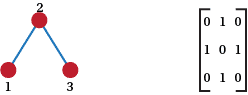## Content

### Alternative representations

In the text so far we have only given the dots and lines version of the representation of graphs. It is also possible to show a list or produce a matrix. There is not much to say about a list. It is just a collection of edges (or single vertices for vertices of degree zero). This would mean that $P$ could be represented as

$12\; ,15\; ,16\; ,23\; , 27\; ,34\; , 38\; , 45\; ,49\; , 510\; ,68\; ,69\; , 79\; ,710,$

where $ab$ represents an edge between $a$ and $b$. As you can see this does not give as much insight into a graph as does the pictorial relation we have used above.

A representation with a little more structure is given by what are known as adjacency matrices. An adjacency matrix is a matrix that shows which vertices are adjacent .For example if we number the rows by numbers 1 to 3 and columns in the same way, Figure 13 shows a graph and its adjacency matrix.A graph in picture form and in matrix form.

It might be obvious, but the top left hand zero shows that 1 is not adjacent to 1, the 1 in the first row and second column shows that 1 is adjacent to 2. We get a 0 in row $a$ and column $b$ if $a$ and $b$ are not adjacent; we get a 1 in row $c$ and column $d$ if $c$ and $d$ are adjacent.

Questions

1. What are the adjacency matrices of the complete graphs and the complete bipartite graphs?
2. How can you see that the adjacency matrix is the graph of a bipartite graph?
3. If $M$ is the adjacency matrix of a graph, what is the adjacency matrix of its complement?
4. What does the adjacency matrix of a graph look like? How can you tell it from a matrix that is not an adjacency matrix of a graph?
5. Let $A$ be the adjacency matrix of a graph. What does $2A$ and $A^2$ look like. What can we tell about the graph from $A^2$? What can you tell about a graph from $A^k$? (Work with some small graphs first to see what pattern develops.)

Next page - Some word problems